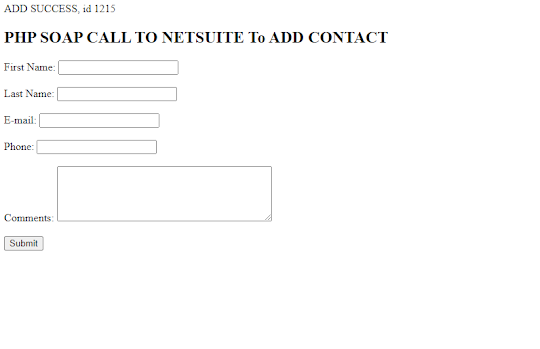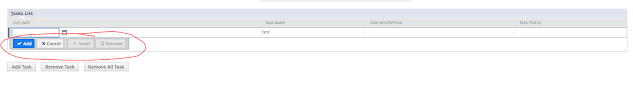## Posts

### Netsuite PHP Toolkit ADD Record Example ( SuiteTalk WebServices)In this example demo we will create a simple php form and send the data to Netsuite to create a contact record through PHP Toolkit for SuiteTalk WebServices. First you need to download the php tool kit and define configuration like connection details in php config file. After that you need to import  require_once '../PHPToolkit/NetSuiteService.php'; In each php file where you want to use the Netsuite Webservices for PHP <!DOCTYPE HTML> <html> <head> <style> .error {color: #FF0000;} </style> </head> <body> <?php require_once '../PHPToolkit/NetSuiteService.php' ; if (\$_SERVER[ "REQUEST_METHOD" ] == "POST" ) { \$contact = new Contact(); \$contact-> entityId =\$_POST[ "firstName" ]. ' ' .\$_POST[ "lastName" ]; \$contact-> firstName = \$_POST[ "firstName" ]; \$contact-> lastName = \$_POST[ "lastName" ]; \$contact-> phone

### Count Total Digits in a Number in Javascript using recursion

In this article we will use recursion to count total digits in a given number function countDigits(n){ if (n == 0 ){ return n; } return 1 + countDigits( parseInt (n/ 10 )); } let sum= countDigits( 999999 ); console.log( "result" + sum); How this works This is  how countDigits function is called 1 + countDigits(99999) returns 1 +4 = 5      1 + countDigits(9999) returns 1 +3 = 4           1 + countDigits(999) returns 1 +2 = 3                1 + countDigits(99) returns 1 +1 = 2                     1 + countDigits(9) returns 1 +0 = 1                          (n == 0 ) returns 0 First function keeps calling by passing argument then function keeps returning and at last will return the final result.

### Sum of digits using Recursion in Javascript.

Recursion in Javascript function sumOfDigits(n){ let num = parseInt(n/ 10 ); let digit = n% 10 ; if (n == 0 ){ return n; } return digit + sumOfDigits(num); } let sum= sumOfDigits( 99999 ); console. log ( "result" + sum); How this works 9 + sumOfDigits(9999) = returns 9 + 36 = 45      9 + sumOfDigits(999) = returns 9 + 27 = 36                9 + sumOfDigits(99) = returns 9 + 18 = 27                          9 + sumOfDigits(9) = returns 9 + 9 = 18                               9 + sumOfDigits(0) = returns 9 So finally it returns 45, which is the sum of digits.

### How to find largest number in an array in Java

All we have to do in this problem is to consider 0 as the largest number then loop all the elements of the array and if any number is greater than the currently set largest number then we will replace the largest number with the new number that is greater and we keep doing this till the end of the array.  class findlargest{ public int largest( int arr[], int n) { int largestNum = 0 ; for ( int i= 0 ; i<arr. length ; i++){ if (arr[i]>largestNum){ largestNum=arr[i]; } } return largestNum; } }

### How to reverse a string in Java

In this problem we are given a string e.g Hello and we need to write a function that will reverse the string and return olleH. In the below function reverseWords we take a string and read each character from the opposite direction then concatenate each charaters to make the string and finally return the string.  class ReverseString { // str: input string public static String reverseWords(String str) { String s= "" ; // Reverse the string str for ( int i=str. length ()- 1 ; i>= 0 ; i--){ char ch=str. charAt (i); s+=ch; } return s; } }

### What are different Sublist Types in Netsuite For suiteletsThere are four sublist types given as follows. INLINEEDITOR EDITOR LIST STATICLIST INLINEEDITOR Sublist   This is a editable sublist where user can add, cancel, insert and remove a line  This is a inlineeditor sublist. A new line is displayed at the bottom of the sublist. fields are displayed below the sublist line  EDITOR Sublist The difference between editor and Inlineeditor sublist is in the way it looks. See UI A new line is added when details are filled and saved. Fields are displayed above the existing sublist lines. LIST Sublist  It has fixed number of lines. You can update existing line but you can not add a line.  STATICLIST Sublist This is a read only sublist which can not be edited in UI and is not available for scripting

### Naming convention for Suitelet fields custpage

In this post we will know how to name fields in suite let. When i am talking about field then it is ID of the field. e.g                                let taskName = form.addField({ id:'custpage_taskname', type: ui.FieldType.TEXT, label:'Task Name' }); in above code id is custpage_taskname Follow the below guidelines to name id Always prefix the field id with custpage_ Do not use any capital letter words in ID  custpage_name  -> This is correct ID custpage_Name -> This is incorrect ID __Name -> This is incorrect ID as it is not prefixed with custpage